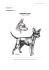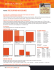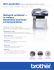# On the Removal of Shadows From Images

## Transcription

On the Removal of Shadows From Images
```1
On the Removal of Shadows From Images
G. D. Finlayson, S. D. Hordley, C. Lu and M. S. Drew
Abstract— This paper is concerned with the derivation of a progression of shadow-free image representations. First we show that
1-d, grey-scale image representation which is illuminant invariant
at each image pixel. We show that as a consequence, images
represented in this form are shadow-free. We then extend this 1-d
representation to an equivalent 2-d, chromaticity representation.
We show that in this 2-d representation, it is possible to re-light
all the image pixels in the same way, effectively deriving a 2-d
we show how to recover a 3-d, full colour shadow-free image
representation by first (with the help of the 2-d representation)
the edge-map of the original image by edge in-painting, and we
propose a method to re-integrate this thresholded edge map, thus
deriving the sought-after 3-d shadow-free image.
Index Terms— Shadow removal, illuminant invariance, reintegration
I. I NTRODUCTION
One of the most fundamental tasks for any visual system
is that of separating the changes in an image which are due
to a change in the underlying imaged surfaces from changes
which are due to the effects of the scene illumination. The
interaction between light and surface is complex and introduces many unwanted artefacts into an image. For example,
changes due to local variation in the intensity or colour of the
illumination all make it more difficult to achieve basic visual
tasks such as image segmentation , object recognition 
and tracking . The importance of being able to separate
illumination effects from reflectance has been well understood
for a long time. For example, Barrow and Tenenbaum 
introduced the notion of “intrinsic images” to represent the
idea of decomposing an image into two separate images:
one which records variation in reflectance, and another which
represents the variation in the illumination across the image.
Barrow and Tenenbaum proposed methods for deriving
such intrinsic images under certain simple models of image
formation. In general however, the complex nature of image
formation means that recovering intrinsic images is an illposed problem. More recently, Weiss  proposed a method
to derive an intrinsic reflectance image of a scene given a
sequence of images of the scene under a range of illumination
conditions. Using many images ensures that the problem is
well-posed, but implies that the application of the method
is quite restricted. The Retinex and Lightness algorithms of
Land  and others , , ,  can also be seen as
an attempt to derive intrinsic reflectance images under certain
G.D. Finlayson and S.D. Hordley are with the University of East Anglia,
Norwich, UK. C. Lu and M.S. Drew are with Simon Fraser University,
restrictive scene assumptions. Specifically, those algorithms
are founded on the premise that scenes are 2-d planar surfaces
constructed from a tessellation of uniform reflectance patches.
In addition, the intensity of illumination across the scene is
assumed to vary only slowly and is assumed to be spectrally
constant. Under these conditions it is possible to distinguish
changes in reflectance from changes in illumination and to
factor the latter out, thus deriving an intrinsic reflectance image
referred to as a lightness image.
Estimating and accounting for the colour of the prevailing
scene illumination is a related problem which has received
much attention , , , , , , , ,
, . In this body of work the focus is not on deriving
intrinsic reflectance images, but rather on obtaining a rendering
of a scene as it would appear when viewed under some standard illumination. Often, these colour constancy algorithms
as they are called, are derived under the same restrictive
conditions as the lightness algorithms, and factors such as
approach to this problem is the so-called illuminant invariant
approach , , , , , , . Instead of
attempting to estimate the colour of the scene illuminant,
illuminant invariant methods attempt simply to remove its
effect from an image. This is achieved by deriving invariant
quantities — algebraic transformations of the recorded image
values — which remain constant under a change of illumination. Methods for deriving quantities which are invariant to one
or more of illumination colour, illumination intensity, shading
and specularities have all been proposed in the literature.
In this paper we consider how we might account for
shadows in an imaged scene: an illumination which has
so far largely been ignored in the body of work briefly
reviewed above. That accounting for the effect of shadows on
colour constancy in images has not received more attention
is somewhat surprising since shadows are present in many
images and can confound many visual tasks. As an example,
consider that we wish to segment the image in Fig. 2a into
distinct regions each of which corresponds to an underlying
surface reflectance. While humans can solve this task easily,
identifying two important regions corresponding to the grass
and the path, such an image will cause problems for a
segmentation algorithm, which will quite likely return at least
three regions corresponding to shadow, grass and path. In
fact, identifying shadows and accounting for their effects is
a difficult problem since a shadow is in effect a local change
in both the colour and intensity of the scene illumination. To
see this, consider again Fig. 2a. In this image, the non-shadow
region is illuminated by light from the sky and also by direct
sunlight, whereas in contrast, the shadow region is lit only by
light from the sky. It follows that to account for shadows we
must be able, in effect, to locally solve the colour constancy
2
problem — that is, identify the colour of the scene illuminant
at each pixel in the scene.
We propose three different shadow-free image representations in this paper. We begin by summarising previous
work ,  which showed that given certain assumptions
about scene illumination and camera sensors it is possible to
solve a restricted colour constancy problem at a single image
pixel. Specifically, given a single triplet of sensor responses it
is possible to derive a 1-d quantity invariant to both the colour
and intensity of the scene illuminant. This in effect provides a
1-d reflectance image which is, by construction, shadow-free.
Importantly, results in this paper demonstrate that applying
the theory to images captured under conditions which fail to
satisfy one or more of the underlying assumptions, still results
in grey-scale images which are, to a good approximation,
shadow-free. Next, we consider how to put some of the colour
back in to the shadow-free representation. We show that there
exists an equivalent 2-d representation of the invariant image
which is also locally illuminant invariant and therefore shadow
free. Furthermore, we show that given this 2-d representation
we can put some illumination back into the scene. That is,
we can re-light all image pixels uniformly (using, e.g., the
illumination in the non-shadow region of the original image)
so that the image remains shadow-free but is closer in colour
to a 2-d representation of the original image. This 2-d image
representation is similar to a conventional chromaticity 
representation (an intensity invariant representation) but with
Finally we show how to recover a full-colour 3-d image
representation which is the same as the original image but
with shadows removed. Here our approach is similar to that
taken in lightness algorithms , , , . In that work
the effects of illumination are factored out by working with an
edge representation of the image, with small edges assumed
to correspond to the slowly changing illumination while large
changes correspond to a change in reflectance. Under these
assumptions, small changes are factored out and the resulting
edge-map is re-integrated to yield an illumination-free lightness image. In our case we also work with an edge-map of
the image but we are concerned with separating shadow edges
from reflectance edges and factoring out the former. To do so
we employ the 2-d shadow-free image we have earlier derived.
We reason that a shadow edge corresponds to any edge
which is in the original image but absent from the invariant
representation, and we can thus define a thresholding operation
to identify the shadow edge. Of course this thresholding
effectively introduces small contours in which we have no edge
information. Thus, we propose a method for in-painting edge
information across the shadow edge. Finally, re-integrating
yields a colour image, equal to the original save for the fact
Before developing the theory of shadow-free images it is
useful to set out some initial assumptions and limitations of
our approach. The derivation of a 1-dimensional image representation, invariant to both illumination colour and intensity,
is founded on a Lambertian model of image formation. That
is, we assume that image pixel values are linearly related to
the intensity of the incident light, and that images are free of
effects such as specularities and interreflections. Furthermore,
the theory is developed under the assumption of an imaging
device with perfectly narrow-band sensors (sensors responsive
to just a single wavelength of light), and we also assume that
our scenes are lit by Planckian illuminants. Of course, not
all of these assumptions will be satisfied for an image of an
arbitrary scene, taken with a typical imaging device. However,
the theory we develop can be applied to any image, and we
discuss, in § II, the effect that departures from the theoretical
case have on the resulting 1-d invariant representation. A more
detailed discussion of these issues can also be found in other
works , . It is also important to point out that, for
some images, the process of transforming the original RGB
representation to the 1-d invariant representation might also
introduce some undesirable artefacts. Specifically, two or more
surfaces which are distinguishable in a 3-d representation,
may be indistinguishable (that is, metameric) in the 1-d
representation. For example, two surfaces which differ only in
their intensity, will have identical 1-d invariant representations.
The same will be true for surfaces which are related by a
change of illumination (as defined by our model). Similar
artefacts can be introduced when we transform an image
from an RGB representation to a 1-d grey-scale representation
since they are a direct consequence of the transformation
from a higher to lower dimensional representation. The 2and 3-dimensional shadow-free representations we introduce
are both derived from the 1-d invariant. This implies that the
assumptions and limitations for the 1-d case also hold true
for the higher dimensional cases. The derivation of the 3d shadow-free image also includes an edge detection step.
Thus, in this case, we will not be able to remove shadows
which have no edges, or whose edges are very ill-defined. In
addition, we point out that edge detection in general is still
an open problem, and the success of our method is therefore
limited by the accuracy of existing edge detection techniques.
Notwithstanding the theoretical limitations we have set out, the
method is capable of giving very good performance on real
images. For example, all the images in Fig. 5 depart from one
or more of the theoretical assumptions and yet the recovered
1-d, 2-d and 3-d representations are all effectively shadowfree.
The paper is organised as follows. In § II we summarise
the 1-d illuminant invariant representation and its underlying
theory. In § III we extend this theory to derive a 2-d representation, and we show how to add illumination back in to this
image, resulting in a 2-d shadow-free chromaticity image. In
§ IV we present our algorithm for deriving the 3-d shadowfree image. Finally in § V we give some examples illustrating
the three methods proposed in this paper, and we conclude the
paper with a brief discussion.
II. 1- D SHADOW FREE IMAGES
Let us begin by briefly reviewing how to derive 1dimensional shadow-free images. We summarise the analysis
given in  for a 3-sensor camera but note that the same
analysis can be applied to cameras with more than three
sensors, in which case it is possible to account for other
3
artefacts of the imaging process (e.g. in  a 4-sensor camera
was considered and it was shown that in this case specularities
could also be removed).
We adopt a Lambertian model  of image formation so
that if a light with a spectral power distribution (SPD) denoted
E(λ, x, y) is incident upon a surface whose surface reflectance
function is denoted S(λ, x, y), then the response of the camera
sensors can be expressed as:
Z
ρk (x, y) = σ(x, y) E(λ, x, y)S(λ, x, y)Qk (λ)dλ
(1)
where Qk (λ) denotes the spectral sensitivity of the kth camera
sensor, k = 1, 2, 3, and σ(x, y) is a constant factor which
denotes the Lambertian shading term at a given pixel — the dot
product of the surface normal with the illumination direction.
We denote the triplet of sensor responses at a given pixel (x, y)
location by ρ(x, y) = [ρ1 (x, y), ρ2 (x, y), ρ3 (x, y)]T .
Given Eq. (1) it is possible to derive a 1-d illuminant
invariant (and hence shadow-free) representation at a single
pixel given the following two assumptions. First, the camera
sensors must be exact Dirac delta functions and second, illumination must be restricted to be Planckian . If the camera
sensitivities are Dirac delta functions, Qk (λ) = qk δ(λ − λk ).
Then Eq. (1) becomes simply:
ρk = σE(λk )S(λk )qk
(2)
where we have dropped for the moment the dependence of ρk
on spatial location. Restricting illumination to be Planckian or,
more specifically, to be modelled by Wien’s approximation to
Planck’s law , an illuminant SPD can be parameterised by
its colour temperature T :
c2
E(λ, T ) = Ic1 λ−5 e− T λ
Summarising Eq. (7) in vector form we have:
1
(8)
e
T
where s is a 2-vector which depends on surface and camera,
but is independent of the illuminant, and e is a 2-vector which
is independent of surface, but which again depends on the
camera. Given this representation, we see that as illumination
colour changes (T varies) the log-chromaticity vector χ0 for
a given surface moves along a straight line. Importantly, the
direction of this line depends on the properties of the camera,
but is independent of the surface and the illuminant.
It follows that if we can determine the direction of illuminant variation (the vector e) then we can determine a 1d illuminant invariant representation by projecting the logchromaticity vector χ0 onto the vector orthogonal to e, which
we denote e⊥ . That is, our illuminant invariant representation
is given by a grey-scale image I:
χ0 = s +
T
I 0 = χ0 e⊥ ,
I = exp(I 0 )
(9)
Without loss of generality we assume that ke⊥ k = 1. Fig. 1a
illustrates the process we have just described. The figure shows
log-chromaticities for four different surfaces (open circles),
for perfect narrow-band sensors under a range of Planckian
illuminants. It is clear that the chromaticities for each surface
fall along a line (dotted lines in the figure) in chromaticity
space. These lines have direction e. The direction orthogonal
to e is shown by a solid line in Fig. 1a. Each log-chromaticity
for a given surface projects to a single point along this line
regardless of the illumination under which it is viewed. These
points represent the illuminant invariant quantity I 0 as defined
in Eq. (9).
(3)
3
Orthogonal Direction
2.5
⊥
e
2
1.5
log(χ2)
where c1 and c2 are constants, and I is a variable controlling
the overall intensity of the light. This approximation is valid
for the range of typical lights T ∈ [2500, 10000]o K. With this
approximation the sensor responses to a given surface can be
expressed as:
1
0.5
0
Lighting Direction
e
−0.5
−1
−3.5
−3
−2.5
−2
−1.5
−1
−0.5
0
0.5
1
1.5
log(χ1)
(a)
(b)
(c)
c
ρk =
− T λ2
k
σIc1 λ−5
k e
S(λk )qk .
(4)
Now let us form band-ratio 2-vector chromaticities χ:
χj =
ρk
, k ∈ {1, 2, 3}, k 6= p, j = 1, 2
ρp
(5)
e.g., for an RGB image, p = 2 means ρp = G, χ1 = R/G,
χ2 = B/G. Substituting the expressions for ρk from Eq. (4)
into Eq. (5) we see that forming the chromaticity co-ordinates
c
− T λ2
χj =
λ−5
k e
k
S(λk )qk
− T λ2p
λ−5
p e
S(λp )qp
c
.
If we now form the logarithm χ0 of χ we obtain:
sk
1
0
χj = log χj = log
+ (ek − ep ), j = 1, 2
sp
T
where sk ≡ λ−5
k S(λk )qk and ek ≡ −c2 /λk .
Fig. 1. (a) An illustration of the 1-d invariant representation, for an ideal
camera and Planckian illumination. (b) The spectral sensitivities of a typical
digital still camera. (c) The log-chromaticities calculated using the sensitivities
from (b) and a set of daylight illuminants.
(6)
(7)
Note that to remove any bias with respect to which colour
channel to use as a denominator,
we can divide by the
√
geometrical mean ρM = 3 RGB in Eq. (5) instead of a
particular ρp and still retain our straight line dependence. Logcolour ratios then live on a plane in 3-space orthogonal to
u = (1, 1, 1)T and form lines exactly as in Fig. 1a .
We have derived this 1-d illuminant invariant representation
under quite restrictive conditions (though the conditions on the
of some conditions on the reflectances ), and it is therefore
reasonable to ask: In practice is the method at all useful? To
answer this question we must first calculate the orthogonal
projection direction for a given camera. There are a number
of ways to do this but the simplest approach is to image a set of
4
reference surfaces (We used a Macbeth Color Checker Chart
which has 19 surfaces of distinct chromaticity) under a series
of n lights. Each surface produces n log-chromaticities which,
ideally, will fall along straight lines. Moreover, the individual
chromaticity lines will also be parallel to one another. Of
course, because real lights may be non-Planckian and camera
sensitivities are not Dirac delta functions we expect there to be
departures from these conditions. Fig. 1b shows the spectral
sensitivities of a typical commercial digital still camera, and in
Fig. 1c we show the log-chromaticity co-ordinates calculated
using these sensitivity functions, the surfaces of a Macbeth
Color Checker and a range of daylight illuminants. It is
clear that the chromaticity co-ordinates do not fall precisely
along straight lines in this case. Nevertheless, they do exhibit
approximately linear behaviour, and so can we solve for the
set of n parallel lines which best account for our data in a least
squares sense . Once we know the orthogonal projection
direction for our camera we can calculate log-chromaticity
values for any arbitrary image. The test of the method is then
whether the resulting invariant quantity I is indeed illuminant
invariant.
Fig. 2 illustrates the method for an image taken with the
camera (modified such that it returns linear output without
any image post-processing) whose sensitivities are shown in
Fig. 1b. Fig. 2a shows the colour image as captured by
the camera (for display purposes the image is mapped to
sRGB  colour space) — a shadow is very prominent.
Figs. 2b,c show the log-chromaticity representation of the
is still clearly visible, highlighting the fact that shadows
represent a change in the colour of the illumination and not
just its intensity. Finally Fig. 2d shows the invariant image (a
function of 2b and 2c) defined by Eq. (9). Visually, it is clear
that the method delivers very good illuminant invariance: the
shadow is not visible in the invariant image. This image is
typical of the level of performance achieved with the method.
Fig. 5 illustrates some more examples for images taken with
a variety of real cameras (with non narrow-band sensors). We
note that in some of these examples, the camera sensors were
unknown and we estimated the illumination direction using
an automatic procedure described elsewhere . In all cases
shadows are completely removed or greatly attenuated.
In other work  we have shown that the 1-d invariant
images are sufficiently illuminant invariant to enable accurate
object recognition across a range of illuminants. In that work,
histograms derived from the invariant images were used as
features for recognition and it is notable that the recognition
performance achieved was higher than that obtained using a
colour constancy approach . It is also notable that the
images used in that work were captured with a camera whose
sensors are far from narrow-band, and under non-Planckian
illuminants. An investigation as to the effect of the shape
of camera sensors on the degree of invariance has also been
carried out . That work showed that good invariance was
achieved using Gaussian sensors with a half bandwidth of up
to 30nm, but that the degree of invariance achievable was
somewhat sensitive to the location of the peak sensitivities of
the sensors. This suggests that there is not a simple relationship
between the shape and width of sensors and the degree of
invariance, so that the suitability of sensors is best judged on
a camera by camera basis. In other work  it has been shown
that it is possible to find a fixed 3×3 linear transform of a given
set of sensor responses so that the 1-d image representation
derived from the transformed sensors has improved illuminant
invariance. In addition, we also note that, for any set of camera
sensors, it is possible to find a fixed 3 × 3 linear transform
which when applied to the sensors brings them closer to the
ideal of narrow-band sensors . Finally, we point out that
in our studies to-date we have not found a set of camera
sensors for which the 1-d representation does not provide a
good degree of illuminant invariance.
(a)
(b)
(c)
(d)
Fig. 2. An example of the 1-d illuminant invariant representation. (a) The
original image; (b) and (c) log-chromaticity representations (χ1 0 and χ2 0 );
(d) the 1-d invariant I.
III. 2- D SHADOW FREE IMAGES
In the 1-d invariant representation described above we
removed shadows but at a cost: we have also removed the
colour information from the image. In the rest of this paper we
investigate how we can put this colour information back in to
the image. Our aim is to derive an image representation which
is shadow-free but which also has some colour information.
We begin by observing that the 1-d invariant we derived
in Eq. (9) can equally well be expressed as a 2-d logchromaticity. Looking again at Fig. 1 we see that an invariant
quantity is derived by projecting 2-d log-chromaticities onto
the line in the direction e⊥ . Equally, we can represent the point
to which a pixel is projected by its 2-d co-ordinates in the logchromaticity space, thus retaining some colour information.
That is, we derive a 2-d colour illumination invariant as:
χ̃0 = Pe⊥ χ0
(10)
where Pe⊥ is the 2 × 2 projector matrix:
Pe⊥ = e⊥ e⊥
T
(11)
5
Pe⊥ takes log-chromaticity values onto the direction orthogonal to e but preserves the resulting quantity as a 2-vector
χ̃0 . The original 1-d invariant quantity I 0 is related to χ̃0 by:
I 0 = χ̃0 · e⊥ .
(12)
To visualise the 2-d invariant image it is useful to express
the 2-d chromaticity information in a 3-d form. To do so,
we write the projected chromaticity 2-vector χ̃0 that lies in a
plane orthogonal to u = (1, 1, 1)T in its equivalent 3-space
co-ordinates ρ̃0 . We do this by multiplying by the 3 × 2 matrix
U T which decomposes the projector onto that plane:
ρ̃0 = U T χ̃0
(13)
where U U T = I − uuT /kuk2 and the resulting ρ̃0 is a 3vector. Note, this transformation is not arbitrary: any 2-d logchromaticity co-ordinates are othogonal to (1, 1, 1) (intensity)
and so we must map 2-d to 3-d accordingly. Finally, by exponentiating Eq (13), we recover an approximation of colour:
ρ̃ = exp(ρ̃0 )
(14)
Note that Eq. (14) is a 3-dimensional representation of 2-d
information: ρ̃ contains no brightness or shading information
and so is still effectively a chromaticity representation. The
usual way to derive an intensity independent representation of
3-d colour is to normalise a 3-d sensor response ρ by the sum
of its elements . We take our 3-d representation into this
form by applying an L1 normalisation:
ρ = {ρ˜1 , ρ˜2 , ρ˜3 }T /(ρ˜1 + ρ˜2 + ρ˜3 )
(15)
This representation is bounded in [0, 1] and we have found
that it has good stability.
An illustration of the method is shown in Fig. 3. Fig. 3a
shows the L1 chromaticity representation r of an image,
with intensity and shading information factored out: r =
{R, G, B}/(R + G + B). It is important to note that in
this representation the shadow is still visible — it represents
a change in the colour of the illumination and not just its
intensity. Fig. 3b shows the illumination invariant chromaticity representation derived in Eqs. (10)-(15) above. Now the
shadow is no longer visible, indicating that the method has
successfully removed the shadow whilst still maintaining some
colour information. Comparing Figures 3a and 3b we see that
must put the illumination back into the representation . Of
course, we don’t want to add illumination back on a pixel-bypixel basis since this would simply reverse what we have just
done and result in an image representation which once again
contains shadows. To avoid this we want to re-light each pixel
uniformly by “adding back” illumination. To see how to do
this, consider again the 2-d chromaticity representation defined
in Eq. (10). In this representation illumination is represented
by a vector of arbitrary magnitude in the direction e:
illumination = χ0E = aE e
(16)
We can put this light back into the illuminant invariant representation defined in Eq. (10) by simply adding the chromaticity
of the light to the invariant chromaticities:
χ̃0 → χ̃0 + χ0E = χ̃0 + aE e
(17)
The colour of the light we put back in is controlled by the value
of aE . To determine what light to add back in we observe that
the pixels in the original image that are brightest, correspond
to surfaces that are not in shadow. It follows then that if we
base our light on these bright pixels then we can use this light
to re-light all pixels. That is, we find a suitable value of aE
by minimising
(18)
kχ0b − (χ̃0b + aE e)k
where χ0b and χ̃0b correspond to the log-chromaticity and
the invariant log-chromaticity of bright (non-shadow) image
pixels. Once we have added the lighting back in this way we
can represent the resulting chromaticity information in 3-d by
applying Eq. (15).
Fig. 3c shows the resulting chromaticity representation with
lighting added back in. Here we found aE by minimising the
term in Eq. (18) for the brightest 1% of pixels in the image.
The colours are now much closer to those in the conventional
chromaticity image (Fig. 3a) but are still not identical. The
remaining difference is due to the fact that when we project
chromaticities orthogonally to the illuminant direction we
remove illumination, as well as any part of a surface’s colour
which is in this direction. This part of the object colour is not
easily put back into the image. Nevertheless, for many surfaces
the resulting chromaticity image is close to the original,
Fig. (5) shows this shadow-free chromaticity representation
for a variety of different images. In all cases, shadows are
successfully removed.
IV. 3- D SHADOW- FREE IMAGES
(a)
(b)
(c)
Fig. 3. (a) A conventional chromaticity representation. (b) The 2-d invariant
representation (χ̃). (c) The 2-d invariant with lighting added back in.
the colours in the two images are quite different. This is because the representation in Fig. 3b has had all its illumination
removed and thus it is in effect an intrinsic reflectance image.
To recover a colour representation closer to that in Fig. 3b we
The 2-d chromaticity representation of images is often very
the value of a chromaticity representation. However, there is
still room for improvement. Chromaticity images lack shading
and intensity information and are also unnaturally coloured.
In some applications an image which is free of shadows, but
which is otherwise the same as a conventional colour image
would be very useful. In this section we consider how such
an image might be obtained.
6
A. The Recovery Algorithm
Our method for obtaining full-colour shadow removal has
its roots in methods of lightness recovery , , , ,
. Lightness algorithms take as their input a 3-d colour image
and return two intrinsic images: one based on reflectance (the
lightness image) and the other based on illumination. Lightness computation proceeds by making the assumption that
illumination varies slowly across an image whereas changes
in reflectance are rapid. It follows then that by thresholding
a derivative image to remove small derivatives, slow changes
(due, by assumption, to illumination) can be removed. Integrating the thresholded derivative image results in the lightness
intrinsic image.
Importantly, a lightness scheme will not remove shadows
since, although they are a change in illumination, at a shadow
edge the illumination change is fast, not slow. Given their
assumptions, lightness algorithms are unable to distinguish
shadow edges from material edges. However, in our case we
have the original image which contains shadows and we are
able to derive from it 1-d or 2-d images which are shadowfree. Thus by comparing edges in the original and the shadowfree images we can identify those edges which correspond to
a shadow. Modifying the thresholding step in the lightness
algorithm leads to an algorithm which can recover full-colour
shadow-free images. There are two important steps which must
be carefully considered if the algorithm is to work in practice.
First, the algorithm is limited by the accuracy with which
we can identify shadow edges. Second, given the location of
the shadow edges we must give proper consideration to how
this can be used in a lightness type algorithm to recover the
Let us begin by defining the recovery algorithm. We use the
notation ρk (x, y) to denote the grey-scale image corresponding
to a single band of the 3-d colour image. Lightness algorithms
work by recovering an intrinsic image from each of these three
bands separately, and combining the three intrinsic images to
form a colour image. We observe in Eq. (4) that under the
assumption of Dirac delta function sensors, sensor response is
a multiplication of light and surface. Let us transform sensor
responses into log space so that the multiplication becomes an
ρ0k (x, y) = σ 0 (x, y) + E 0 (λk , x, y) + S 0 (λk , x, y) + qk0 (19)
In the original lightness algorithm the goal is to remove
illumination and, as a first step towards this, gradients are
calculated for the log-image:
∂ 0
ρ (x, y)
∇x ρ0k (x, y) =
∂x k
∂ 0
∇y ρ0k (x, y) =
ρ (x, y)
(20)
∂y k
These gradients define edge maps for the log image. Next, a
threshold operator T (·) is defined to remove gradients of small
magnitude:

if k∇i ρ0k (x, y)k < τ
 0
0
T (∇i ρk (x, y)) =

∇i ρ0k (x, y) otherwise
(21)
where i ∈ {x, y} and τ is the chosen threshold value.
In our case the goal is not to remove illumination per se (the
small values in (21) above) but rather we wish only to remove
shadows. In fact we actually want to keep the illuminant
field and re-render the scene as if it were captured under
the same single non-shadow illuminant. To do this we must
do this by modifying the threshold operator defined in (21).
In principle, identifying shadows is easy: we look for edges
in the original image which are not present in the invariant
representation. However, in practice the procedure is somewhat
more complicated than this. For now, let us assume that we
have identified the shadow edge and leave a discussion of how
we find it to the next section. Let us define a function qs (x, y)

 1 if (x, y) is a shadow edge
qs (x, y) =
(22)

0 otherwise
We can then remove shadows in the gradients of the log image
using the threshold function TS (·):

if qs (x, y) = 1
 0
TS (∇i ρ0k , qs (x, y)) =
(23)

∇i ρ0k otherwise
where again i ∈ {x, y}. That is, wherever we have identified
that there is a shadow edge we set the gradient in the logimage to zero, indicating that there is no change at this
point (which is true for the underlying reflectance). After
thresholding we obtain gradients where sharp changes are
indicative only of material changes: there are no sharp changes
due to illumination and so shadows have been removed.
We now wish to integrate edge information in order to
recover a log-image which does not have shadows. We do
this by first taking the gradients of the thresholded edge maps
we have just defined to form a modified (by the threshold
operator) Laplacian of the log-image:
∇2TS ρ0k (x, y) =
∇x TS (∇x ρ0k (x, y), qs (x, y))
+∇y TS (∇y ρ0k (x, y), qs (x, y))
(24)
Now, let us denote the shadow-free log-image which we wish
to recover as ρ̃0 (x, y) and equate its Laplacian to the modified
Laplacian we have just defined:
∇2 ρ̃0k (x, y) = ∇2TS ρ0k (x, y)
(25)
Equation (25) is the well known Poisson equation. The
shadow-free log-image can be calculated via:
−1 2 0
ρ˜k 0 (x, y) = ∇2
∇TS ρk (x, y)
(26)
However, since the Laplacian is not defined at the image
boundary without boundary conditions, we must specify these
for uniqueness. Blake  made use of Neumann boundary
conditions, in which the normal derivative of the image is
specified at its boundary. Here we use homogeneous Neumann
conditions: the directional derivative at the boundary is set to
zero.
There are two additional problems with recovering ρ˜k 0 (x, y)
according to Eq. (26) caused by the fact that we have removed
7
shadow edges from the image. First, because we have modified
the edge maps by setting shadow edges to zero, we can no
longer guarantee that the edge map we are integrating satisfies
the integrability condition. For the edge map to be integrable
the following condition should be met (cf. ):
∇y ∇x ρ0k (x, y) = ∇x ∇y ρ0k (x, y)
(27)
The second problem is caused by the fact that to ensure
shadows are effectively removed, we must set to zero, edges
in quite a large neighbourhood of the actual shadow edge.
As a result edge information pertaining to local texture in the
neighbourhood of the shadow edge is lost and the resulting
(shadow-free) image is unrealistically smooth in this region.
To avoid this problem, rather than simply setting shadow edges
to zero in the thresholding step, we apply an iterative diffusion
process which fills in the derivatives across shadow edges,
bridging values obtained from neighbouring non-shadow edge
pixels. We also deal with the problem of integrability at
this stage by including a step at each iteration to enforce
integrability, as proposed in .
This iterative process is detailed below where t denotes
artificial time:
1. Initialisation, t = 0, calculate:
(∇x ρ0k (x, y))t → TS (∇x ρ0k (x, y), qs (x, y))
(∇y ρ0k (x, y))t → TS (∇y ρ0k (x, y), qs (x, y))
the Discrete Fourier Transform (DFT). The projection step
deriving Z(u, v) follows , but for a forward-difference
operator.
4. if k(∇x ρ0 )t − (∇x ρ0 )t−1 k + k(∇y ρ0 )t − (∇y ρ0 )t−1 k ≥ ,
t → t + 1, goto 2.
where defines the stopping criterion.
Finally, we then solve the Poisson equation (26) using a
final round of enforcing integrability by projection as above,
with the re-integrated image given by
ρ̃0k (x, y) = F −1 [Z(u, v)]
(28)
We actually operate on an image four times the original
size, formed by symmetric replication in x and y, so as to
enforce periodicity of the data for the DFT and homogeneous
Neumann boundary conditions.
Eq. (28) recovers ρ̃0k (x, y) up to an unknown constant
of integration. Exponentiating ρ̃0k (x, y), we arrive at the reconstructed grey-scale image ρ̃k (x, y) (up to an unknown
multiplicative constant). Solving (26) for each of the three
colour bands results in a full colour image ρ̃ = {ρ̃1 ρ̃2 ρ̃3 }T
To fix the unknown multiplicative factors, we apply a mapping to each pixel which maps the brightest pixels (specifically,
the 0.005-percentile of pixels ordered by brightness) in the
recovered image to the corresponding pixels in the original
image.
2. Update shadow edge pixels (i, j):
(∇x ρ0k (i, j))t →
(∇x ρ0k (i − 1, j))t−1 + (∇x ρ0k (i, j − 1))t−1
To complete the definition of the recovery algorithm we
must specify how to identify shadow edges. The essential idea
is to compare edge maps of the original image to those derived
from an invariant image, and to define a shadow edge to be any
edge in the original which is not in the invariant image. We
could start by calculating edge maps as simple finite difference
(∇x ρ0k (i + 1, j))t−1 + (∇x ρ0k (i, j + 1))t−1
(∇y ρ0k (i, j))t →
(∇y ρ0k (i − 1, j))t−1 + (∇y ρ0k (i, j − 1))t−1
+(∇y ρ0k (i + 1, j))t−1 + (∇y ρ0k (i, j + 1))t−1
3. Enforce integrability by projection onto integrable edge
map , and integrate:
Fx (u, v) =
F[∇x ρ0k ],
Fy (u, v) =
F[∇y ρ0k ],
ax = e2πiu/N − 1 , ay = e2πiv/M − 1,
Z(u, v) =
a∗x Fx (u, v) + a∗y Fy (u, v)
, ρ0 (0, 0) = 0,
|ax |2 + |ay |2
(∇x ρ0 )t = F −1 [ax Z] , (∇y ρ0 )t = F −1 [ay Z]
where image size is M × N and F[·] denotes the Fourier
Transform. Here we use forward-difference derivatives
{−1, 1}T , {−1, 1} corresponding to the ax , ay above in the
Fourier domain: i.e., the Fourier transform of a derivative
∇x Z in the spatial domain corresponds to multiplication by
ax (u) in the Fourier domain — this result simply follows
by writing ρ0 (n + 1) − ρ0 (n) in terms of Fourier sums in
∇x ρI (x, y) = ρI (x, y) ⊗ {−1, 0, 1}T /2
∇y ρI (x, y) = ρI (x, y) ⊗ {−1, 0, 1}/2
(29)
where ρI (x, y) is the intensity image, taken here as the L1
norm of the original image: ρI = (1/3)(ρ1 + ρ2 + ρ3 ). Unfortunately, as Fig. 4a illustrates, finite differencing produces
non-zero values at more locations than those at which there
are true edges. Thus, while in the example in Fig. 4a the edges
of the road and the shadow are clear, so too are many edges
due to the texture of the imaged surfaces as well as noise in
the image. Obtaining the true edges in which we are interested
from these edge maps is non-trivial, as evidenced by the large
literature on edge detection (see  for a review).
For a more careful approach, we begin by applying a
smoothing filter (specifically the Mean-Shift algorithm proposed in ) to both the original image and the 2-d invariant
image derived by exponentiating the invariant log image. This
has the effect of suppressing features such as noise and high
frequency textures so that in subsequent processing fewer
8
spurious edges are detected. Then, we replace simple differencing by the Canny edge detector , returning estimates
for the strength of horizontal and vertical edges at each image
location:
˜ x ρi (x, y)k = Cx [ρi (x, y)]
k∇
(30)
˜ y ρi (x, y)k = Cy [ρi (x, y)]
k∇
edge map is defined

1







qs (x, y) =







0
where Cx [·] and Cy [·] denote the Canny (or any other wellbehaved) operators for determining horizontal and vertical
edges respectively.
We determine an edge map for the invariant image in
a similar way, first calculating horizontal and vertical edge
strengths for each channel of the 2-d invariant image:
where τ1 , τ2 , and τ3 are thresholds whose values are parameters in the recovery algorithm. As a final step, we employ
a morphological operation (specifically, two dilations) on the
binary edge map to “thicken” the shadow edges:
˜ x χk (x, y)k = Cx [χk (x, y)]
k∇
where ⊕ denotes the dilation operation and D denotes the
structural element, in this case the 8-connected set. This
dilation has the effect of filling in some of the gaps in
the shadow edge. Fig. 4d illustrates a typical example of a
recovered shadow edge map qs (x, y). It is clear that even after
the processing described, the definition of the shadow edge is
imperfect: there are a number of spurious edges not removed.
However, this map is sufficiently accurate to allow recovery
of the shadow-free image shown in Fig. (4e) based on the
integration procedure described above.
(31)
˜ y χk (x, y)k = Cy [χk (x, y)]
k∇
The edge maps from the two channels are then combined by
a max operation:
˜ x χ̃(x, y)k = max (Cx [χ̃1 (x, y)], Cx [χ̃2 (x, y)])
k∇
(32)
˜ y χ̃(x, y)k = max (Cy [χ̃1 (x, y)], Cy [χ̃2 (x, y)])
k∇
where max(·, ·) returns the maximum of its two arguments
at each location (x, y). Figs. 4b and 4c show the resulting
(a)
(b)
(d)
(c)
(e)
Fig. 4. (a) An edge-map obtained using simple finite differencing operators.
(b) Edges obtained using the Canny operator on the Mean-Shifted original
image. (c) Edges obtained using the Canny operator on the Mean-Shifted 2-d
colour image.
edge maps for the original image (calculated by (30)) and
the invariant image (calculated by (31)-(32)). While still not
perfect, the real edges in each image are now quite strong and
we can compare the two edge maps to identify shadow edges.
We use two criteria to determine whether or not a given
edge corresponds to a shadow. First, if at a given location the
original image has a strong edge but the invariant image has a
weak edge, we classify that edge as a shadow edge. Second, if
both the original image and the invariant image have a strong
edge, but the orientation of these edges is different, then we
as:
˜ i k > τ1 & k∇χk
˜
< τ2
if k∇ρ
˜ x ρi k
or kk∇
˜ y ρi k −
∇
˜ x χk k∇
˜ y χk k∇
> τ3
(33)
otherwise
qs (x, y) → (qs (x, y) ⊕ D) ⊕ D
(34)
V. D ISCUSSION
We have introduced three different shadow-free image representations in this paper: a 1-d invariant derived from first
principles based on simple constraints on lighting and cameras,
a 2-d chromaticity representation which is equivalent to the 1-d
representation but with some colour information retained and,
finally, a 3-d full colour image. Fig. 5 shows some examples
of these different representations for a number of different
images. In each example all three representations are shadowfree. The procedure for deriving each of the three representations is automatic, but there are a number of parameters
which must be specified. In all cases we need to determine
the direction of illumination change (the vector e discussed
in § II). This direction can be found either by following the
calibration procedure outlined in § II above or, as has recently
been proposed  by using a procedure which determines
the illuminant direction from a single image of a scene having
obtained based on the latter calibration procedure. In addition
to the calibration step, in the 2-d representation we also have
a parameter to control how much light is put back in to the
image. We used the procedure described in § III to determine
this parameter for the examples in Fig. 5.
Recovering the 3-d representation is more complex and
there are a number of free parameters in the recovery algorithm. As a first step the original full-colour images were
processed using the mean shift algorithm which has two free
parameters: a spatial bandwidth parameter which was set to
16 (corresponding to a 17 × 17 spatial window), and a range
parameter which was set to 20. The process of comparing the
two edge maps is controlled by three thresholds: τ1 , τ2 and
τ3 . τ1 and τ2 relate to the edge strengths in the original and
9
the invariant image, respectively. We chose values of τ1 = 0.4
and τ2 = 0.1 after the gradient magnitudes have been scaled to
a range [0, 1]. Our choice for these parameters is determined
by the hysteresis step in the Canny edge detection process.
τ3 controls the difference in the orientation between edges
in the original image and those in the invariant. Edges are
classified into one of eight possible orientations, but by taking
advantage of symmetry we need consider only four of them.
So τ3 is set equal to π/4. These parameters were fixed for all
images in Fig. 5 and, although the recovered shadow edge is
not always perfect, the resulting shadow-free image is, in all
cases, of good quality. We note however, that the algorithm in
its current form will not deliver perfect shadow-free images
in all cases. In particular, images with complex shadows, or
diffuse shadows with poorly defined edges will likely cause
problems for the algorithm. However, the current algorithm
is robust when shadow edges are clear, and we are currently
investigating ways to improve the algorithm’s performance on
the more difficult cases. In addition, it is possible for the
method to misclassify some edges in the original image as
in intensity, an edge detector will find an edge at the border
of these two surfaces. However, in the 1-d invariant image
intensity differences are absent, and so no edge will be found
in this case. Thus, the edge between the two surfaces will
wrongly be classified as a shadow edge. Indeed, the fifth
example in Fig. 5 exhibits such behaviour: the boundary
between the painted white line on the road surface, and the
road surface itself, is not fully recovered, because the two
surfaces (paint and road) differ mainly in intensity. A similar
problem can arise if adjacent surfaces are related by a colour
change in the direction in which illumination changes. Here
again, an edge will be found in the original image, but will
be absent from the invariant images. The examples in Fig. 5
(and the many other images we have processed) suggest that
such problems arise only infrequently in practice. However, in
future work we intend to investigate ways to overcome these
problems.
In summary, we conclude that the approach to shadow
removal proposed in this paper yields very good performance.
In all three cases (1-d, 2-d and 3-d) the recovered images
are of a good quality and we envisage that they will be of
practical use in a variety of visual tasks such as segmentation,
image retrieval, and tracking. As well, the method raises
the possibility of enhancing commercial photography such as
portraiture.
R EFERENCES
 G. J. Klinker, S. A. Shafer, and T. Kanade, “A physical approach to color
image understanding,” International Journal of Computer Vision, vol. 4,
pp. 7–38, 1990.
 M. J. Swain and D. H. Ballard, “Color Indexing,” International Journal
of Computer Vision, vol. 7, no. 1, pp. 11–32, 1991.
 H. Jiang and M. Drew, “Tracking objects with shadows.” in ICME’03:
International Conference on Multimedia and Expo, 2003.
 H. Barrow and J. Tenenbaum, “Recovering intrinsic scene characteristics
from images,” in Computer Vision Systems, A. Hanson and E. Riseman,
Eds. Academic Press, 1978, pp. 3–26.
 Y. Weiss, “Deriving intrinsic images from image sequences,” in ICCV01.
IEEE, 2001, pp. II: 68–75.
 E. H. Land, “Lightness and retinex theory,” Journal of the Optical Society
of America, A, vol. 61, pp. 1–11, 1971.
 B. K. Horn, “Determining Lightness from an Image,” Computer Graphics
and Image Processing, vol. 3, pp. 277–299, 1974.
 A. Blake, “On Lightness Computation in the Mondrian World,” in
Proceedings of the Wenner-Gren Conference on Central & Peripheral
Mechanisms in Colour Vision. MacMillan, New York, 1983, pp. 45–59.
 G. Brelstaff and A. Blake, “Computing lightness,” Pattern Recognition
Letters, vol. 5, pp. 129–138, 1987.
 A. Hurlbert, “Formal connections between lightness algorithms,” Journal
of the Optical Society of America, A, vol. 3, no. 10, pp. 1684–1693, 1986.
 E. H. Land, “The Retinex Theory of Color Vision,” Scientific American,
pp. 108–129, 1977.
 L. T. Maloney and B. A. Wandell, “Color constancy: a method for
recovering surface spectral reflectance,” Journal of the Optical Society
of America, A, vol. 3, no. 1, pp. 29–33, 1986.
 G. Buchsbaum, “A spatial processor model for object colour perception,”
Journal of the Franklin Institute, vol. 310, pp. 1–26, 1980.
 D. Forsyth, “A Novel Algorithm for Colour Constancy,” International
Journal of Computer Vision, vol. 5, no. 1, pp. 5–36, 1990.
 G. D. Finlayson, S. D. Hordley, and P. M. Hubel, “Color by correlation:
A simple, unifying framework for color constancy,” IEEE Transactions
on Pattern Analysis and Machine Intelligence, vol. 23, no. 11, pp. 1209–
1221, 2001.
 D. H. Brainard and W. T. Freeman, “Bayesian Method for Recovering
Surface and Illuminant Properties from Photosensor Responses,” in Proceedings of the IS&T/SPIE Symposium on Electronic Imaging Science &
Technology, vol. 2179, 1994, pp. 364–376.
 V. Cardei, “A neural network approach to colour constancy,” Ph.D.
dissertation, Simon Fraser Univ., School of Computing Science, 2000.
 K. Barnard, “Practical colour constancy,” Ph.D. dissertation, Simon
Fraser Univ., School of Computing Science, 2000.
 S. Tominaga and B. A. Wandell, “Standard surface-reflectance model
and illuminant estimation,” Journal of the Optical Society of America, A,
vol. 6, no. 4, pp. 576–584, 1996.
 H.-C. Lee, “Method for computing scene-illuminant chromaticity from
specular highlights,” in Color, Glenn E. Healey and Steven A. Shafer and
Lawrence B. Wolff, Ed. Jones and Bartlett, Boston, 1992, pp. 340–347.
 Th. Gevers and H. M. G. Stokman, “Classifying color transitions into
shadow-geometry, illumination highlight or material edges,” in International Conference on Image Processing, 2000, pp. 521–525.
 B. V. Funt and G. D. Finlayson, “Color Constant Color Indexing,” IEEE
Transactions on Pattern Analysis and Machine Intelligence, vol. 17, no. 5,
pp. 522–529, 1995.
 G. Finlayson, S. Chatterjee, and B. Funt, “Color angular indexing,” in
The Fourth European Conference on Computer Vision (Vol II). European
Vision Society, 1996, pp. 16–27.
 T. Gevers and A. Smeulders, “Color based object recognition,” Pattern
Recognition, vol. 32, pp. 453–464, 1999.
 M. Stricker and M. Orengo, “Similarity of color images,” in SPIE Conf.
on Storage and Retrieval for Image and Video Databases III, vol. 2420,
1995, pp. 381–392.
 D. Berwick and S. W. Lee, “A chromaticity space for Specularity, Illumination color- and illumination pose-invariant 3-D object recognition,”
in Sixth International Conference on Computer Vision. Narosa Publishing
House, 1998.
 G. Finlayson, B. Schiele, and J. Crowley, “Comprehensive colour image
normalization,” in eccv98, 1998, pp. 475–490.
 G. Finlayson and S. Hordley, “Color constancy at a pixel,” J. Opt. Soc.
Am. A, vol. 18, no. 2, pp. 253–264, 2001, also, UK Patent application
no. 0000682.5. Under review, British Patent Office.
 J. A. Marchant and C. M. Onyango, “Shadow invariant classification
for scenes illuminated by daylight,” Journal of the Optical Society of
America, A, vol. 17, no. 12, pp. 1952–1961, 2000.
 R. Hunt, The Reproduction of Colour, 5th ed. Fountain Press, 1995.
 J. L. N. J. Romero, J. Hernandez-Andres and E. Valero, “Testing spectral
sensitivity of sensors for color invariant at a pixel,” in 2nd Computer
Graphics, Imaging and Vision Conference. IS&T/SID, April 2004.
 G. Finlayson and M. Drew, “4-sensor camera calibration for image
in ICCV’01: International Conference on Computer Vision. IEEE, 2001,
pp. 473–480.
 B. K. Horn, Robot Vision. MIT Press, 1986.
 G. Wyszecki and W. Stiles, Color Science: Concepts and Methods,
Quantitative Data and Formulas, 2nd ed. New York:Wiley, 1982.
 G. D. Finlayson, D. M. S, and C. Lu., “Intrinsic images by entropy
minimisation,” in ECCV04, 2004.
10
 M. H. Brill and G. Finlayson, “Illuminant invariance from a single
reflected light,” Color Research and Application, vol. 27, pp. 45–48, 2002.
 M. Stokes, M. Anderson, S. Chandrasekar, and R. Motta, “A
standard default color space for the internet - srgb,” 1996,
http://www.color.org/sRGB.html.
 B. Funt, K. Barnard, and L. Martin, “Is machine colour constancy good
enough?” in 5th European Conference on Computer Vision. Springer,
June 1998, pp. 455–459.
 M. Drew, C. Chen, S. Hordley, and G. Finlayson, “Sensor transforms
for invariant image enhancement,” in Tenth Color Imaging Conference:
Color, Science, Systems and Applications. Society for Imaging Science
& Technology (IS&T)/Society for Information Display (SID) joint conference, 2002, pp. 325–329.
 G. D. Finlayson, M. S. Drew, and B. V. Funt, “Spectral Sharpening:
sensor transformations for improved color constancy,” Journal of the
Optical Society of America, A, vol. 11, no. 5, pp. 1553–1563, 1994.
 M. S. Drew, G. D. Finlayson, and S. D. Hordley, “Recovery of
chromaticity image free from shadows via illumination invariance,” in
Workshop on Color and Photometric Methods in Computer Vision. IEEE,
2003, pp. 32–39.
 B. Funt, M. Drew, and M. Brockington, “Recovering shading from color
images,” in ECCV-92: Second European Conference on Computer Vision,
G. Sandini, Ed. Springer-Verlag, May 1992, pp. 124–132.
 R. Frankot and R. Chellappa, “A method for enforcing integrability
in shape from shading algorithms,” IEEE Trans. Patt. Anal. and Mach.
Intell., vol. 10, pp. 439–451, 1988.
 R. Jain, R. Kasturi, and B. Schunck, Machine Vision. McGraw-Hill,
1995.
 D. Comaniciu and P. Meer, “Mean shift analysis and applications,” in
Proceedings of the 7th International Conference on Computer Vision.
IEEE, 1999, pp. 1197–1203.
 J. Canny, “A computational approach to edge detection,” IEEE Trans.
Patt. Anal. Mach. Intell., vol. 8, pp. 679–698, 1986.
G. D. Finlayson obtained his BSc in Computer Science from the University
of Strathclyde (Glasgow, Scotland) in 1989. He then pursued his graduate
education at Simon Fraser University (Vancouver, Canada) where he was
awarded his MSc and PhD degrees in 1992 and 1995 respectively. From
August 1995 until September 1997, Dr Finlayson was a Lecturer in Computer
Science at the University of York (York, UK) and from October 1997 until
August 1999 he was a Reader in Colour Imaging at the Colour & Imaging
institute, University of Derby (Derby, UK). In September 1999. he was
appointed a Professor in the School of Computing Sciences, University of
East Anglia (Norwich, UK).
S. D. Hordley obtained his BSc in Mathematics from the University of
Manchester (UK) in 1992 and an MSc degree in Image Processing from
Cranfield Institute of Technology (UK) in 1996. Between 1996 and 1999 he
studied at the University of York (UK) and the University of Derby (UK) for
a PhD in Colour Science. In September 2000 he was appointed a Lecturer in
the School of Computing Sciences at the University of East Anglia (Norwich,
UK).
C. Lu is a Ph.D. candidate in the School of Computing Science at Simon
Fraser University in Vancouver, Canada. He received his undergraduate education in Computer Applications at the University of Science and Technology
in Beijing, China, and received his Masters degree in the School of Computing
Science at Simon Fraser University. His research interests include image and
video indexing, computer vision, and color processing for digital photography.
M. S. Drew is an Associate Professor in the School of Computing Science
at Simon Fraser University in Vancouver, Canada. His background education
is in Engineering Science, Mathematics, and Physics. His interests lie in the
fields of multimedia, computer vision, image processing, color, photorealistic
computer graphics, and visualization. He has published over 80 refereed
papers in journals and conference proceedings. Dr. Drew is the holder of
a U.S. Patent in digital color processing.
11
Fig. 5.
Some example images. From left to right: original image, 1-d invariant representation, 2-d representation, and recovered 3-d shadow-free image.
```

### modern designs - Resene Paints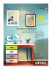### Palace of Justice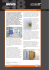### Untitled - System 24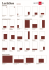### nueva in nudes - Market America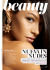### ES14-OTDR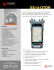### Digital Marketing Trends 2015 - Dundalk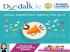### palette of the past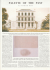### (Standard FCI finální)